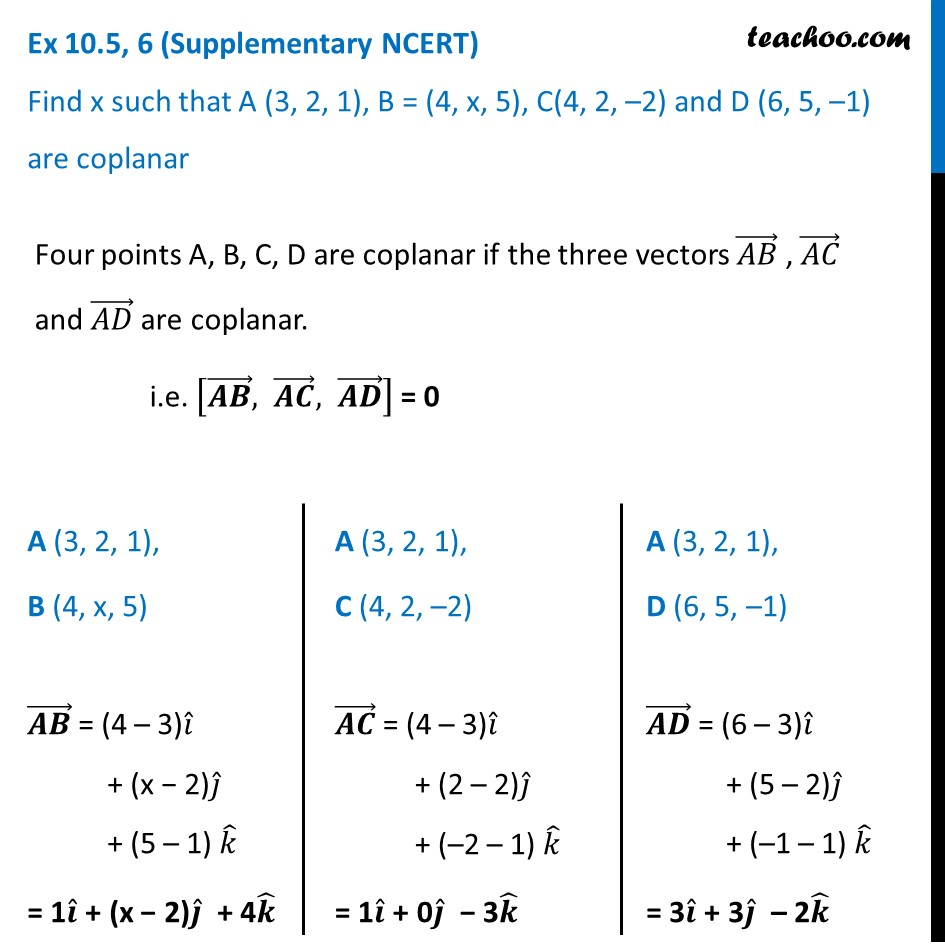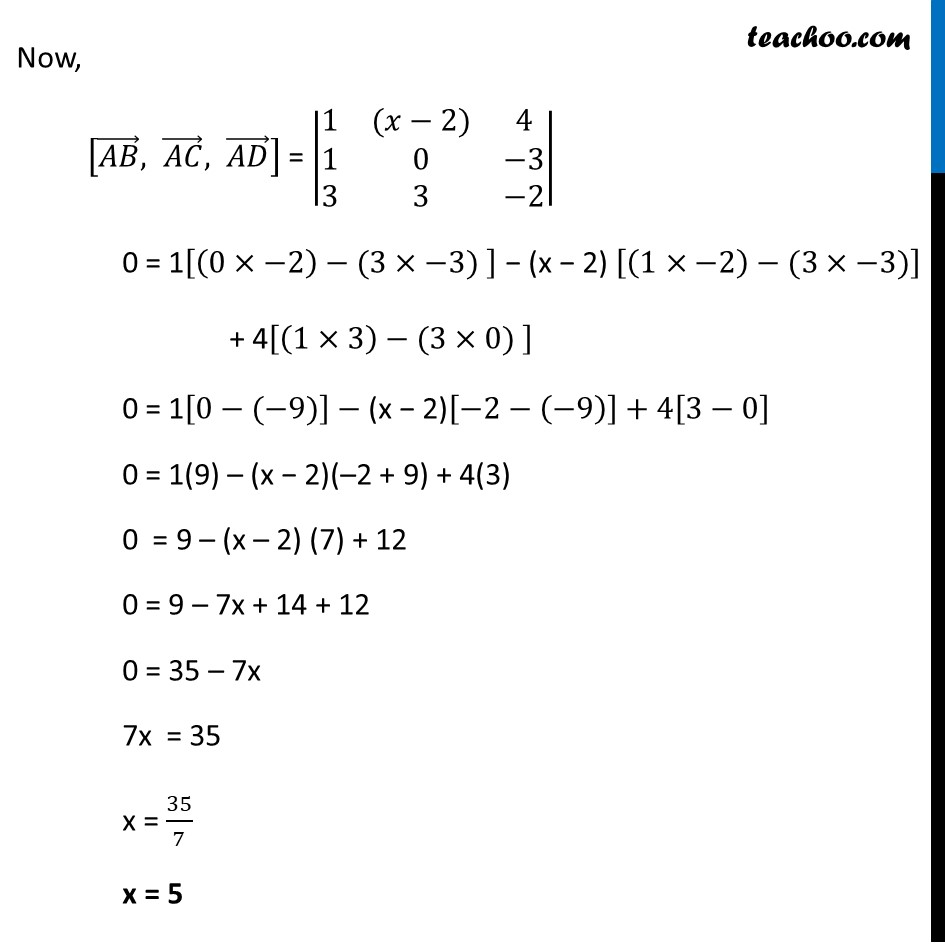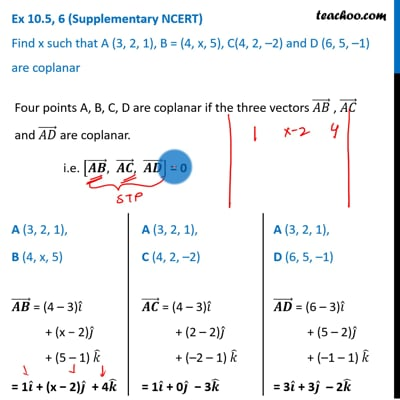Ex 10.5 (Supplementary NCERT)

Chapter 10 Class 12 Vector Algebra
Serial order wiseThis video is only available for Teachoo black users

Introducing your new favourite teacher - Teachoo Black, at only ₹83 per month

### Transcript

Ex 10.5, 6 (Supplementary NCERT) Find x such that A (3, 2, 1), B = (4, x, 5), C(4, 2, –2) and D (6, 5, –1) are coplanar Four points A, B, C, D are coplanar if the three vectors (𝐴𝐵) ⃗ , (𝐴𝐶) ⃗ and (𝐴𝐷) ⃗ are coplanar. i.e. [(𝑨𝑩) ⃗, (𝑨𝑪) ⃗, (𝑨𝑫) ⃗ ] = 0 A (3, 2, 1), B (4, x, 5) (𝑨𝑩) ⃗ = (4 – 3)𝑖 ̂ + (x − 2)𝑗 ̂ + (5 – 1) 𝑘 ̂ = 1𝒊 ̂ + (x − 2)𝒋 ̂ + 4𝒌 ̂ A (3, 2, 1), C (4, 2, –2) (𝑨𝑪) ⃗ = (4 – 3)𝑖 ̂ + (2 – 2)𝑗 ̂ + (–2 – 1) 𝑘 ̂ = 1𝒊 ̂ + 0𝒋 ̂ − 3𝒌 ̂ A (3, 2, 1), D (6, 5, –1) (𝑨𝑫) ⃗ = (6 – 3)𝑖 ̂ + (5 – 2)𝑗 ̂ + (–1 – 1) 𝑘 ̂ = 3𝒊 ̂ + 3𝒋 ̂ – 2𝒌 ̂ Now, [(𝐴𝐵) ⃗, (𝐴𝐶) ⃗, (𝐴𝐷) ⃗ ] = |■8(1&(𝑥−2)&4@1&0&−3@3&3&−2)| 0 = 1[(0×−2)−(3×−3) ] − (x − 2) [(1×−2)−(3×−3)] + 4[(1×3)−(3×0) ] 0 = 1[0−(−9)]−"(x − 2)" [−2−(−9)]+4[3−0] 0 = 1(9) – "(x − 2)"(–2 + 9) + 4(3) 0 = 9 – (x – 2) (7) + 12 0 = 9 – 7x + 14 + 12 0 = 35 – 7x 7x = 35 x = 35/7 x = 5